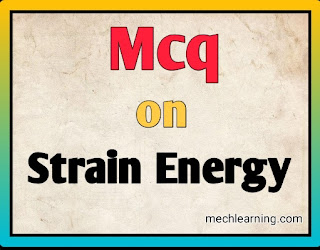# Mcq on strain energy

The Mechanical engineering, is plays a important branch in the today's Technology so let's learn about Concept of Mcq on strain energy - machine design that helps to modernize our society.Mcq on strain energy

## Multiple choice questions MCQ on strain energy is as follows:-

1. When The energy stored in a body then strain within elastic limit is called as...
A. Proof resilience
B. Modulus of resilience
C. Resilience
D. Strain energy

2. The Total strain energy stored in a body is called as...
A. Proof resilience
B. Modulus of resilience
C. Resilience
D. Impact energy

3. The Proof resilience is defined as maximum strain energy stored in the body is called as proof resilience.
A. True
B. False

4. Total Strain energy that can be stored in a body is called as resilience.
A. True
B. False

5. The strain energy stored in the body when load is applied gradually is....
a) σE/V
b) σE2/V
c) σV2/E
d) σV2/2E

6. The maximum Energy stored in body is called as strain energy.
A. True
B. False

7. The Proof resilience per unit it's volume of the materials is called as strain energy.
A. True
B. False

8. The strain energy stored in the body to uniform stress (s) of volume the (V) is....
a) s2V/2E
b) sV/E
c) sV2/E
d) sV/2E

9. The Total strain energy stored in body is called as proof resilience.
A. True
B. False

10. The proof resilience per unit volume of materials is known as...
A. Modulus of elasticity
B. Modulus of resilience
C. Strain energy
D. Modulus of rigidity

11. The unit of modulus of resilience is....
A. N/mm3
B. N/mm2
C. N/mm
D. N/m

12. The Strain energy per unit volume for the solid is q2 / 4G.
A. True
B. False

13. The unit of resilience is Newton (N).
A. True
B. False

14. The energy stored after fracture is called as...
A. Modulus of elasticity
B. Modulus of resilience
C. Strain energy
D. Toughness

15. The Strain energy is described as work done.
A. True
B. False

16. The strain energy stored in the body and when the load is applied suddenly then strain energy is.....
a) 2 × σE/V
b) σE2/V
c) σV2/E
d) 2 × σV2/2E

A. Thrice
B. Twice
C. Equal
D. None of the above

18. The Strain energy stored in a body upto proportionality limit is called as proof resilience.
A. True
B. False

Previous Post
Next Post